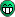# what is interpolation?

wiki.synfig.org/wiki/Waypoint#Interpolation

Does it mean the way animation is handled? Is there some other article that explains it in plain english?

Since all tutorials use TCB Smooth interpolation, I’m guessing thats the one most practical. I get constant, which stops the animation.

Hi omar1!
I’ll try to write a small resume of the concepts here:
Synfig document (sifz) is made of layers. Layers are created based on its parameters. Each parameter is a Value type (real, string, integer, boolean, vector, list, etc.). Values can be Constant or Animated. One constant parameter doesn’t have any waypoint on its timeline and the value is always the same, regardless the current document time value. Animated Values do have a list of Waypoints. A Waypoint is the joint of four data:

1. Time: the time where the waypoint is placed.
2. Value: when the document time is the same than the waypoint’s time, the parameter has the value defined in the waypoint.
3. ‘In’ interpolation: It is an integer that can have one of those values: Clamped (new default), TCB, Linear, Ease (in or out), Constant.
4. ‘Out’ interpolation. Same as ‘In’.

When the document time is not the time of a waypoint, Synfig calculates an interpolated value for that animated parameter. Say that you have one time ‘T’ that is after one certain waypoint ‘A’ and before other waypoint ‘B’.
The value of the parameter at time ‘T’ depends on:

1. The relative position between A’s time, B’s time and ‘T’.
2. The values at waypoint A and B.
3. The value of interpolation ‘Out’ at A and the value of interpolation ‘In’ at B

So, for example:
Waypoint A
Time: 2 seconds
Value: 10.0 units
In: what ever
Out: Linear

Waypoint A
Time: 4 seconds
Value: 15.0 units
In: Linear
Out: whatever

If you place the time cursor at time T=3 seconds you’ll get a value of:
V=10.0 units + [(15.0 units -10.0 units)/(4 s - 2 seconds)]*(T-2 seconds)
So it is V = 12.5 units.

Each combination of Out/In interpolations gives a math function that defines the parameter between the two waypoints. You shouldn’t take care of the maths, just look the Curves panel and see how does the parameter variation along the time is.

I hope it helped.
-G

Thank you, that cleared it up a bit.

I also played around with a simple ball animation, two instances of a ball (one white, the other blue) bouncing on two different layers, and tried out different interpolation in the middle bounce waypoint on one. I see now how it affects the animation and coordinates.

Hello omar1I had you made an image with color circles, but in the meantime, you solved the problemHere it is anywayhttp://skias.free.fr/images/interpolation.png